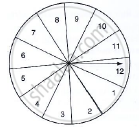# A Game of Chance Consists of Spinning an Arrow Which is Equally Likely to Come to Rest Pointing to One of the Number, 1, 2, 3, ..., 12 as Shown in Fig. Below. What is the Probability that It Will Point To 10? - Mathematics

A game of chance consists of spinning an arrow which is equally likely to come to rest pointing to one of the number, 1, 2, 3, ..., 12 as shown in Fig. below. What is the probability that it will point to 10?#### Solution

Total no. of possible outcomes = 12 {1, 2, 3,…., 12}

Let E ⟶ event of pointing 10

No. favourable outcomes = 1 {10}

P(E) ="No.of favorable outcomes"/"Total no.of possible outcomes"=1/12

Concept: Probability - A Theoretical Approach
Is there an error in this question or solution?

#### APPEARS IN

RD Sharma Class 10 Maths
Chapter 16 Probability
Exercise 16.1 | Q 21.1 | Page 21